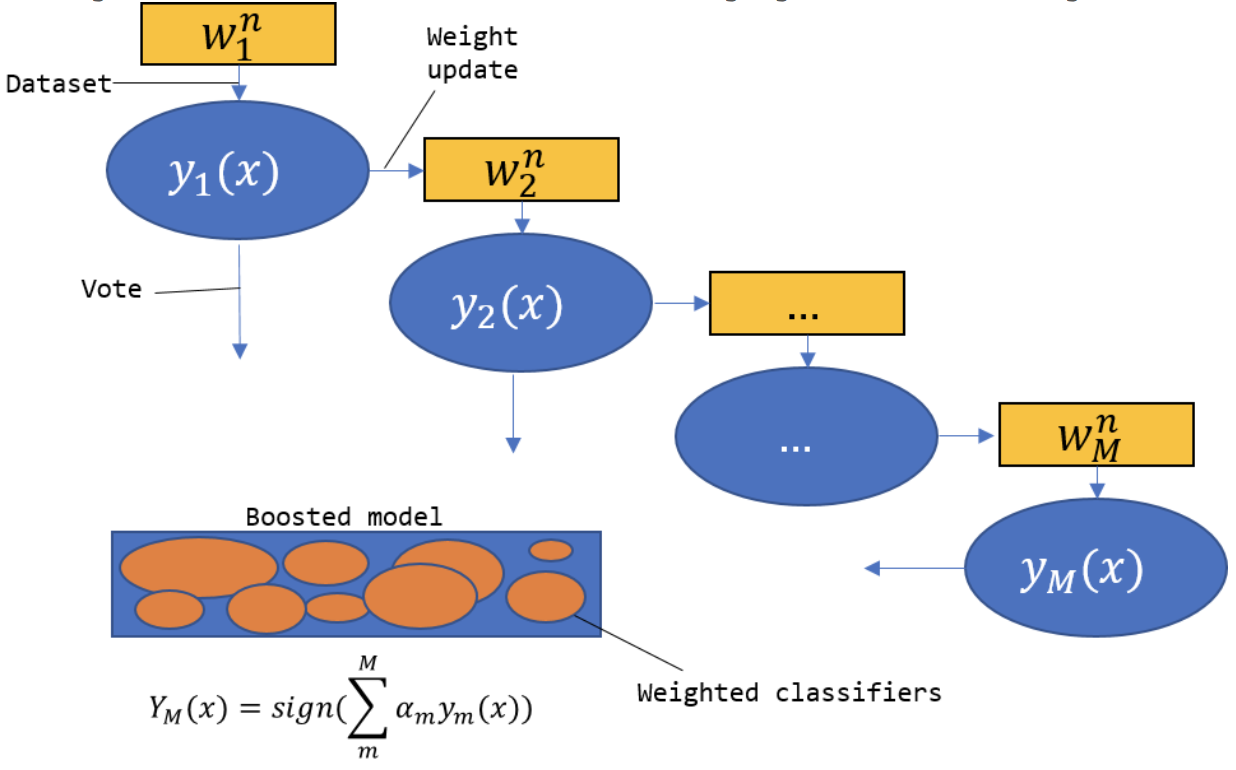# 1. 概述

## 1.1 集成学习

$$H(x) = sign(\sum_{i=1}^M h_i(x)) \tag{1.1.1}$$

$$P(H(x) \neq f(x)) \leq exp(- \frac 1 2 M (1-2\epsilon)^2) \tag{1.1.2}$$

1. 基学习器之间存在强依赖关系，一系列基学习器需要串行生成，代表算法是Boosting
2. 基学习器之间不存在强依赖关系，一系列基学习器可并行生成，代表算法是Bagging随机森林

## 1.2 Boosting

Boosting指的是一类集成方法，其主要思想就是将弱的基学习器提升(boost)为强学习器。具体步骤如下:

1. 先用每个样本权重相等的训练集训练一个初始的基学习器；
2. 根据上轮得到的学习器对训练集的预测表现情况调整训练集中的样本权重(例如提高被错分类的样本的权重使之在下轮训练中得到更多的关注), 然后据此训练一个新的基学习器；
3. 重复2直到得到 $M$ 个基学习器，最终的集成结果是 $M$ 个基学习器的组合。

## 2.1 基本思想

1. 如何调整每一轮的训练集中的样本权重？
2. 如何将得到的 $M$ 个组合成最终的学习器？

1. 提高上一轮被错误分类的样本的权值，降低被正确分类的样本的权值；
2. 线性加权求和。误差率小的基学习器拥有较大的权值，误差率大的基学习器拥有较小的权值。## 2.2 算法步骤

$${(x_1,y_1),(x_2,y_2),…,(x_N,y_N)}$$

1. 初始化样本的权重
$$D_1=(w_{11}, w_{12},…w_{1N}), w_{1i}=\frac 1 N, i = 1,2…N \tag{2.2.1}$$
2. 对$m = 1,2,…M$,重复以下操作得到 $M$ 个基学习器:
(1) 按照样本权重分布 $D_m$ 训练数据得到第 $m$ 个基学习器 $G_m(x)$:
$$G_m(x): \mathcal{X} \to {-1, +1}$$
(2) 计算 $G_m(x)$ 在加权训练数据集上的分类误差率:
$$e_m = \sum_{i=1}^NP(G_m(x_i) \neq y_i)=\sum_{i=1}^N w_{mi} I(G_m(x_i) \neq y_i) \tag{2.2.2}$$
上式中 $I(\cdot)$ 是指示函数，考虑更加周全的AdaBoost算法在这一步还应该判断是否满足基本条件(例如生成的基学习器是否比随机猜测好), 如果不满足，则当前基学习器被抛弃，学习过程提前终止。
(3) 计算 $G_m(x)$ 的系数(即最终集成使用的的基学习器的权重):
$$\alpha_m = \frac 1 2 log \frac {1-e_m} {e_m} \tag{2.2.3}$$
(4) 更新训练样本的权重
$$D_{m+1}=(w_{m+1,1}, w_{m+1,2},…w_{m+1,N}) \tag{2.2.4}$$
$$w_{m+1, i} = \frac{w_{mi}} {Z_m} exp(-\alpha_my_iG_m(x_i)) ,i=1,2,…N \tag{2.2.5}$$
其中 $Z_m$ 是规范化因子，目的是为了使 $D_{m+1}$ 的所有元素和为1。即
$$Z_m=\sum_{i=1}^N w_{mi} exp(-\alpha_my_iG_m(x_i)) \tag{2.2.6}$$
3. 构建最终的分类器线性组合
$$f(x) = \sum_{i=1}^M \alpha_m G_m(x) \tag{2.2.7}$$
得到最终的分类器为
$$G(x) = sign(f(x))=sign(\sum_{i=1}^M \alpha_m G_m(x)) \tag{2.2.8}$$

## 3.1 前向分步算法

$$f(x) = \sum_{i=1}^M \alpha_m G_m(x) \tag{3.1.1}$$

$$min \sum_{i=1}^N L(y_i, f(x)) \iff min \sum_{i=1}^N L(y_i, \sum_{i=1}^M \alpha_m G_m(x)) \tag{3.1.2}$$

$$f_m(x)= f_{m-1}(x) + \alpha_mG_m(x) \tag{3.1.3}$$

$$min \sum_{i=1}^N L(y_i, f_{m-1}(x) + \alpha_mG_m(x)) \tag{3.1.4}$$

$$L(y, f(x)) = exp(-yf(x))$$

$$\underset{\alpha_m,G_m}{argmin} \sum_{i=1}^N exp[-y_i(f_{m-1}(x) + \alpha_mG_m(x))] \tag{3.2.1}$$

$$w_{m,i} = exp[-y_i f_{m-1}(x)] \tag{3.2.2}$$

$$\underset{\alpha_m,G_m}{argmin} \sum_{i=1}^N w_{m,i}exp(-y_i\alpha_mG_m(x)) \tag{3.2.3}$$

1. 对任意的 $\alpha_m > 0$, 求 $\hat{G}_m(x)$：
$$\hat{G}_m (x) = \underset{G_m}{argmin} \sum_{i=1}^N w_{m,i} I(y_i \neq G_m(x_i)) \tag{3.2.4}$$

上式将指数函数换成指示函数是因为前面说的指数损失函数和0/1损失函数是一致等价的。

式子 $(3.2.4)$ 所示的优化问题其实就是AdaBoost算法的基学习器的学习过程，即2.2节的步骤2(1)，得到的 $\hat{G}_m(x)$ 是使第 $m$ 轮加权训练数据分类误差最小的基分类器。

2. 求解 $\hat{\alpha}_m$ ：
将式子 $(3.2.3)$ 中的目标函数展开
\begin{aligned} \sum_{i=1}^N w_{m,i}exp(-y_i\alpha_mG_m(x)) &= \sum_{y_i=G_m(x_i)} w_{m,i}e^{- \alpha} + \sum_{y_i \neq G_m(x_i)}w_{m,i}e^{\alpha} \\\\ & = (e^{\alpha} - e^{-\alpha}) \sum_{i=1}^N w_{m,i} I(y_i \neq G_m(x_i)) + e^{-\alpha} \sum_{i=1}^N w_{m,i} \end{aligned} \tag{3.2.5}

注：为了简洁，上式子中的$\hat{G}_m(x)$ 被略去了 $\hat{\cdot}$ ， $\alpha_m$ 被略去了下标 $m$ ，下同

将上式对 $\alpha$ 求导并令导数为0，即
$$(e^{\alpha} + e^{-\alpha}) \sum_{i=1}^N w_{m,i} I(y_i \neq G_m(x_i)) - e^{-\alpha} \sum_{i=1}^N w_{m,i} = 0 \tag{3.2.6}$$
解得
$$\hat{\alpha}_m = \frac 1 2 log \frac {1-e_m} {e_m} \tag{3.2.7}$$
其中, $e_m$ 是分类误差率：
$$e_m = \frac {\sum_{i=1}^N w_{m,i} I(y_i \neq G_m(x_i)} {\sum_{i=1}^N w_{mi}} \tag{3.2.8}$$
如果式子 $(3.2.8)$ 中的 $w_{mi}$ 归一化成和为1的话那么式 $(3.2.8)$ 也就和2.2节式 $(2.2.2)$ 一模一样了，进一步地也有上面的 $\hat{\alpha}_m$ 也就是2.2节的 $\alpha_m$ 。
最后来看看每一轮样本权值的更新，由 $(3.1.3)$ 和 $(3.2.2)$ 可得
$$w_{m+1,i} = w_{m,i} exp[-y_i \alpha_m G_{m}(x)] \tag{3.2.9}$$
如果将上式进行归一化成和为1的话就和与2.2节中 $(2.2.5)$ 完全相同了。